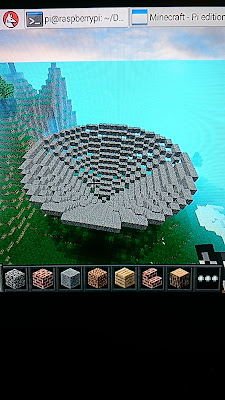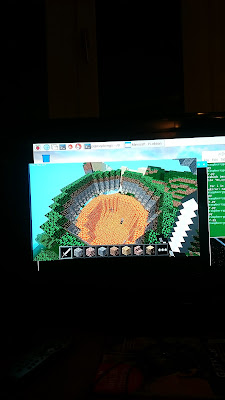## Circles in Minecraft?

Using Minecraft Pi, you can write code to place blocks. This means, in theory, you can code objects you could never build accurately - like giant circles.

I had a go at some maths around this in a spreadsheet using the formula for a circle

where x and y are coordinates.

It came out like this:

Which is circular if nothing else.

I don't like the gaps near the axes but with some clever programming (which I'll have to google!) I reckon eventually I could get rid of them.

#### Trig Method

I realised the numbers above follow 2 overlapping sine waves, so a quick search revealed this:

"A circle can be defined as the locus of all points that satisfy the equations
x = r cos(t)    y = r sin(t)
where x,y are the coordinates of any point on the circle, r is the radius of the circle and
t is the parameter - the angle subtended by the point at the circle's center."
from  http://www.mathopenref.com/coordparamcircle.html (there's an interactive applet)

So  by carefully choosing the angular interval 't' you should be able to put blocks precisely where you want them in the circle.

Here's my first go in the spreadsheet:

You can see there are still gaps and the numbers in the columns are all over the place. Think the trig functions are working in radians but I entered angles in degrees.

To plot more points, I'd have to reduce the interval in column 1 to give more points between 0 and 2.

Imagine that in Minecraft!

I've a Pi 3 that I've just set up to run headless, so I'll move onto that next.....

The Results!nested loop of expanding rising circles. Feels like an amphitheatre inside.Simple cylinder - circles on top of circlesThis one was weird - a cylinder with different block types in each layer. Unfortunately 2 of them were lava and water.One massive circle.

The code for these:

``````
from mcpi.minecraft import Minecraft
import math

mc = Minecraft.create()
#get players position
x,y,z=mc.player.getPos()

#move out of the way, there's something coming
mc.player.setPos (x,y+50,z)

for q in range (1,4):  #nested loop for when you want multiple circles
for i in range(0,r*6):  #sets how many blocks comprise the shape
j = float(i)/(r*3)  #gives max j of 2 (to give  2*pi radians for trig))

blockXPos = r*math.cos(j*math.pi)  #sets x coordinate of block
blockZPos = r*math.sin(j*math.pi)  #sets z coordinate of block

mc.setBlock(blockXPos+x,y+q,blockZPos+z,1) #places a block in the circle

```
```

You could use code like this to plot other mathematical functions. Even 3D ones:Its something called a quadric surface:Something about that makes me feel like I've earned a glass of wine!